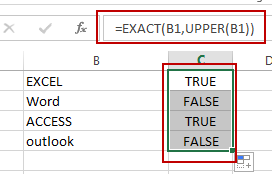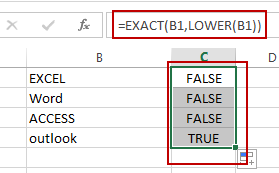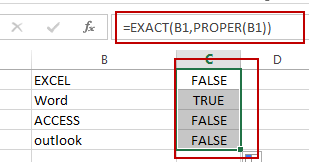# How to Check If a Cell is Uppercase, Lowercases or Capitalize in Excel

This post will guide you how to check if a cell is uppercase in Excel 2013/2016. How do I identify all letters in cells are lowercases or capitalize in Excel. How to change letters to uppercase, lowercases or capitalize first letter for all words in cells in Excel.

## Check If All Letters are Uppercase

If you want to check if all letters in cells are uppercase, you can use a formula based on the EXACT function and the UPPER function. Like this:

`=EXACT(B1,UPPER(B1))`

Type this formula into a blank cell and press Enter key to apply this formula. If All letters are uppercases, and it returns TRUE. Otherwise, it returns FALSE. Then drag the AutoFill Handle down to other cells to apply this formula.Let’ see how this formula works:

The UPPER function will convert all letters to uppercase. And then pass the result to EXACT function as its second argument. And the EXACT function will compare if two values are equal. If equal, returns TRUE. Otherwise, returns FALSE.

## Check If All Letters are Lowercase

If you need to identify all characters in cells are lowercase, you can use a formula based on the LOWER function and the EXACT function. Like this:

`=EXACT(B1,LOWER(B1))`

Type this formula into cell C1, and press Enter key to apply this formula. If all letters are lowercases, and it returns TRUE. Otherwise, it returns FALSE. Then drag the AutoFill Handle down to other cells to apply this formula.The LOWER function converts all letters to lowercase.

## Check If the First Letters are capitalized

If you want to check if the first character in each word is capitalized, you can use a formula based on the EXACT function and the PROPER function. Like this:

`=EXACT(B1,PROPER(B1))`

Type this formula into cell C1, and press Enter key to apply this formula. If all the first letters are capitalized, and it returns TRUE. Otherwise, it returns FALSE. Then drag the AutoFill Handle down to other cells to apply this formula.The PROPER function will capitalize the first letter in each word in Cell.

### Related Functions

• Excel UPPER function
The Excel UPPER function converts all characters in text string to uppercase.The UPPER function is a build-in function in Microsoft Excel and it is categorized as a Text Function.The syntax of the UPPER function is as below:= UPPER (text)…
• Excel LOWER function
The Excel LOWER function converts all characters in a text string to lowercase.The syntax of the LOWER function is as below:= LOWER  (text)…
• Excel Proper Function
The Excel PROPER function capitalizes the first character in each word of a text string and set other characters to lowercase. The syntax of the PROPER function is as below:= PROPER  (text)…
• Excel EXACT function
The Excel EXACT function compares if two text strings are the same and returns TRUE if they are the same, Or, it will return FALSE.The syntax of the EXACT function is as below:= EXACT (text1,text2)…

Related Posts

If Cell Begins with One of Three Supplied Characters

If you want to test values to see if they begin with some given specific characters like “x”, ”y”, or “z”, you can create a formula with COUNTIF and SUM functions to return results. EXAMPLE You can see “TRUE” or ...

Fix #N/A Error For VLOOKUP From Another Sheet

This post will show you how to fix the #N/A error why it occurs when you extract values from another sheet using VLOOKUP function in Excel 2016,2013,2010 or other Excel versions. How can you correct a #N/A error in VLOOKUP ...

How To Convert Text to Upper Cases(Using VBA) in Excel

This post will show you how to switch from lower case to upper case in Excel. and I am going to show you two different ways of converting text to upper cases using formula or VBA macro in Excel 2013,Excel ...

How to Average Only Positive or Negative Numbers of a Range

Suppose both positive numbers and negative numbers exist in a table. If we want to know the average of only positive numbers in this table, we can create a formula to get average of all positive numbers with all negative ...

Sort Positive Numbers and Negative Numbers by Absolute Values

If both positive numbers and negative numbers exist in the same column, when sorting them by absolute values, we can sort them with the help of ABS function and helper column. In this article, we will show you the way ...

Get Employee Information by VLOOKUP

VLOOKUP is one of the key functions among all lookup & reference functions in Excel. Today, in this article, we will show you the way to apply VLOOKUP to retrieve employee information. I hope this article will help you in ...

VLOOKUP with Two Lookup Tables

VLOOKUP is one of the key functions among all lookup & reference functions in Excel. Today we will show you the application of VLOOKUP function when there are two lookup tables. EXAMPLE Table1 and table2 record the rates of Y2020 ...

VLOOKUP with Multiple Lookup Values

VLOOKUP is one of the key functions among all lookup & reference functions in Excel. It can scan and retrieve data from a static or dynamic table based on your lookup value. It can perform approximate match or exact match ...

VLOOKUP Data by Date

VLOOKUP is one of the key functions among all lookup & reference functions in Excel. It can scan and retrieve data from a static or dynamic table based on your lookup value. It can perform approximate match or exact match ...

VLOOKUP – Retrieve Data from Another Workbook

VLOOKUP is one of the key functions among all lookup & reference functions in Excel. It can scan and retrieve data from a static or dynamic table based on your lookup value. It can perform approximate match or exact match ...

Sidebar